#### You may also like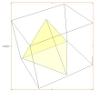### A Mean Tetrahedron

Can you number the vertices, edges and faces of a tetrahedron so that the number on each edge is the mean of the numbers on the adjacent vertices and the mean of the numbers on the adjacent faces?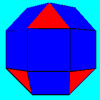### Rhombicubocts

Each of these solids is made up with 3 squares and a triangle around each vertex. Each has a total of 18 square faces and 8 faces that are equilateral triangles. How many faces, edges and vertices does each solid have?### Icosian Game

This problem is about investigating whether it is possible to start at one vertex of a platonic solid and visit every other vertex once only returning to the vertex you started at.

# Triangles to Tetrahedra

##### Age 11 to 14Challenge Level

Students attending a masterclass at the Thomas Deacon Academy in Peterborough tried to work on this problem systematically. Here are examples of how they went about it.

I think their ideas are excellent and give an insight into how you might make a convincing argument that you have all possibilities. Well done to you all for trying to describe your approaches to this problem.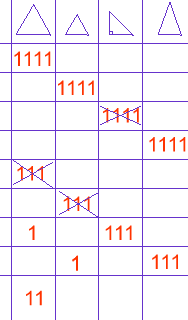Susannah, Adam, Maria and Erin adopted an approach like the one illustrated in this table. Can you see how they have been systematic and continue their argument? Thanks for this neat idea .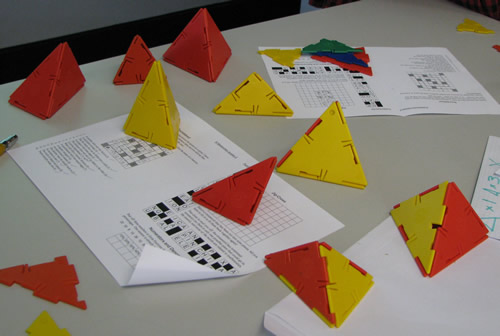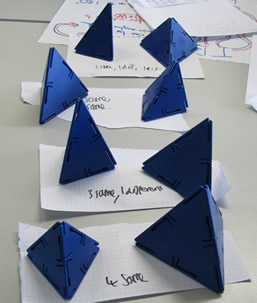Stephen, Hugh, Daniel and Deepak, adopted a similar approach. Firstly, they identified and coded each of the four triangles, identifying the long and the short sides of each. Then they considered sets of four triangles in a systematic way. Can you see how they worked systematically from the table below:

 All the same E,E,E,E SE,SE,SE,SE I,I,I,I R,R,R,R - no 3 and 1 E,E,E,SE- no E,E,E,I - no E,E,E,R-no SE,SE,SE,E - no SE,SE,SE,I -no SE,SE,SE,R-no I,I,I,E - no I,I,I,SE I,I,I,R - no R,R,R,E R,R,R,SE - no R,R,R,I - no 2 and 2 ... ... ...

Emily, Clara, Lizzie and Kieran chose an approach using some further ideas to help them be more efficient:
1. There are only two lengths of sides (long, as in the length of the sides of the large equilateral triangle and the hypotenuse of the right-angled triangle; short, as in the length of the sides of the small equilateral triangle and the short side, or base, of the isosceles triangle).
2. There must be an even number of long and and even number of short sides in the sets of four triangles that form the tetrahedron if the triangles fit together.
3. The even number of sides have to be spread across the triangles, for example four shorts are no good if three of them are all on a small equilateral triangle.
We coded the triangles as follows:
Big equilaterals - EB
Small equilaterals - ES
Isosceles - I
Right-angled - R

We tabulated all possibilities and quickly crossed out those without an even number of long and an even number of short sides. We then considered whether we could actually make those left, like this (I have not included the whole of their table but can you see how they were systematically listing all the possibilities ):

 Even number of longs and shorts/does it work? I I I I YES/YES EB EB EB EB YES/YES ES ES ES ES YES/YES R R R R YES/NO EB EB EB I NO EB EB EB ES NO EB EB EB R NO I I I ES YES/YES I I I R NO I I I EB NO R R R I NO R R R ES NO R R R EB YES/YES ES ES ES I YES/NO ES ES ES EB NO ES ES ES R NO EB EB I I YES/YES EB EB I ES YES/NO
and so on...

Chris, Rana, Nabil and Indrajeet took a quite different approach, using tree diagrams. Here is one of the diagrams they produced. They needed to do a tree diagram starting with each of the triangles and look carefully for duplications .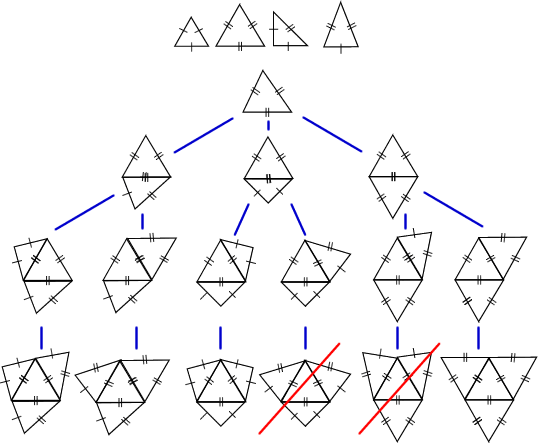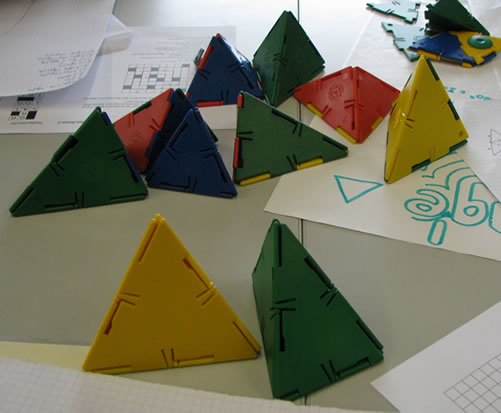Other solutions we have received included one from Mark Johnson, who found tetrahedra that use these triangles:
1 small equilateral 3 isosceles
1 big equilateral 3 right-angled
2 big equilateral 2 isosceles
4 small equilateral
4 big equilateral
2 small equilateral 2 right-angled
2 isosceles 1 right-angled 1 big equilateral
2 isosceles 2 right-angled (two different arrangements produce tetrahedra that are reflections of each other )

Yanqing from Lipson Community College can add one more to Mark's list :
1 small equilateral 1 isosceles 2 right-angled

Altogether we have found a total of 10 different tetrahedra.